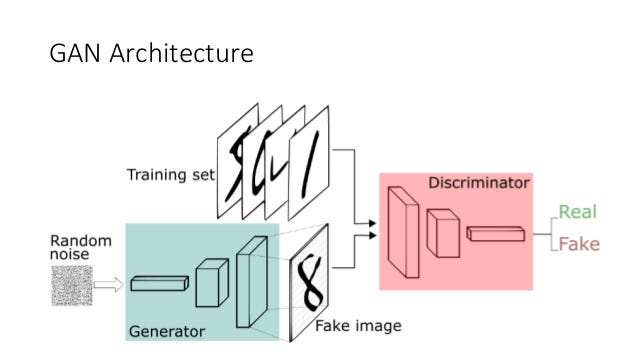# Learning Generative Adversarial Networks (GANs)# Learning Generative Adversarial Networks (GANs)

GANs were introduced in a paper by Ian Goodfellow and other researchers at the University of Montreal in 2014.

# What is GAN?

A generative adversarial network (GAN) is a type of model in neural network that offer a lots of potential in the world of machine learning. In GAN there are two neural networks: first is generative network and second is discriminative network. So the main concept behind this project is the generative adversarial network. GAN is about creating stuff and this is hard to compare other deep leaning fields. The main focus of GAN is to generate data from scratch. As we see early GAN composes of two networks the generator and the discriminator.

Generative adversarial networks (GANs) are deep neural net architectures included of two nets, pitting one against the other.

Facebook’s AI research director Yann LeCun called adversarial training “the most interesting idea in the last 10 years in ML.”

GANs’ potential is very huge because they can learn to mimic any data. So use of GAN we create worlds similar to our own in any domain: image, anime, news anchor, speech.

# Generative vs. Discriminative Algorithms

To understand GANs, we need to know how generative algorithms work as well as how discriminative algorithms are sententious, so the work of discriminative algorithms tries to classify the data.
A standard example of this scenario is email, given all words in an email what discriminator do is predict whether the message is spam or not spam. In this example, spam is one of the labels and words of the email are features that compose the input data. If we expressed this problem is mathematical, so the label is called y and the feature is called x. The formulation p (y|x) is used to mean “the probability of y given x”.

The main question a generative algorithm tries to answer is: they assuming this email is spam while the discriminative model cares about the relations between feature(x) and label(y).
So if we think about generative algorithms is that they do opposite compare to the discriminator. Instead of predicting a label they predict the features given a certain label.
Best way to distinguish generative from the discriminative like this:

• Discriminative models learn the range between classes
• Generative models model the distribution of individual classes

# How GANs Work

As we know these algorithms belong to the field of unsupervised learning
Generative Adversarial Networks are composed of two models:

The first model is called a Generator and its target to generate new data similar to the real one. Generator can create data and discriminator is checked whether the data is real or fake.

And the second model is called a Discriminator. This model’s goal is to recognize if an input data is real or fake — belongs to the original dataset- or if it’s fake generated by the generator. So discriminator is like a police which tries to detect work is real or fake.

When training begins, the generator produces fake data, and the discriminator quickly learns to tell that it’s fake.

After training, the generative model can then be used to create new plausible samples on demand.

GANs have very specific use cases and it can be difficult to understand these use cases when getting started.

# How do these models interact?

In the original paper which proposed this framework, it can be thought of the Generator as having an adversary, the Discriminator. So that means generator needs to learn how to do operations as well as create data in such a way discriminator isn’t able to distinguish between the real and fake or it as fake anymore. The competition between these two models is what improves their knowledge until the generator is creating realistic data

# Fundamental step to Train a GAN

I. Sample a noise set data and a real data set. Each with size m.

II. Train the Discriminator on this data.

III. Sample a different noise subset with size m.

IV. Train the generator on this data.

V. Repeat from step 1.

Here are the steps a GAN takes:

• So the generator takes in random number and retunes an image.
• The generated image is compared to the actual dataset and fed into the discriminator.
• Then discriminator takes both real and fake images and return probabilities and numbers between 0 and 1 like logistic regression. 1 is representing real and 0 is representing fake.

So we have two feedback loop:

• The discriminator is in a feedback loop with the ground truth of the images.
• The generator is in a feedback loop with the discriminator.

Here’s a picture of the whole system:

As we see both are dynamic. Discriminator network is a standard convolutional network that can categorize the image fed to it, and the generator is inverse convolutional network.

Objective function

GAN is defined as a minimax game with the following objective function.